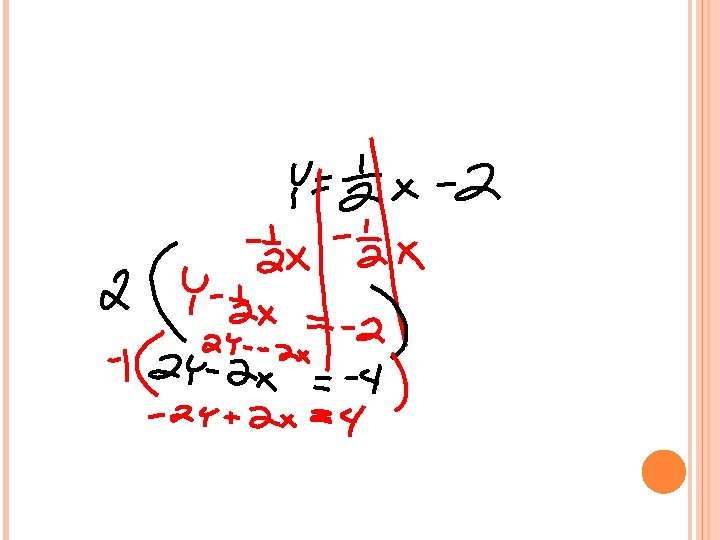# STANDARD FORM STANDARD FORM For standard form equations

• Slides: 22STANDARD FORM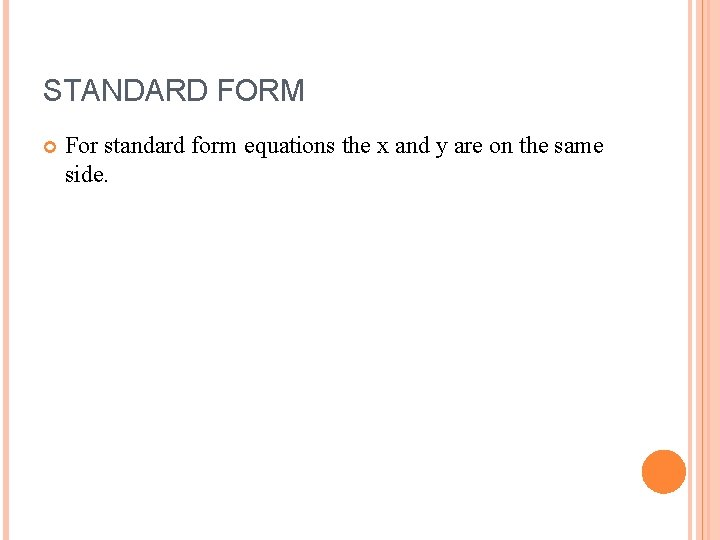STANDARD FORM For standard form equations the x and y are on the same side.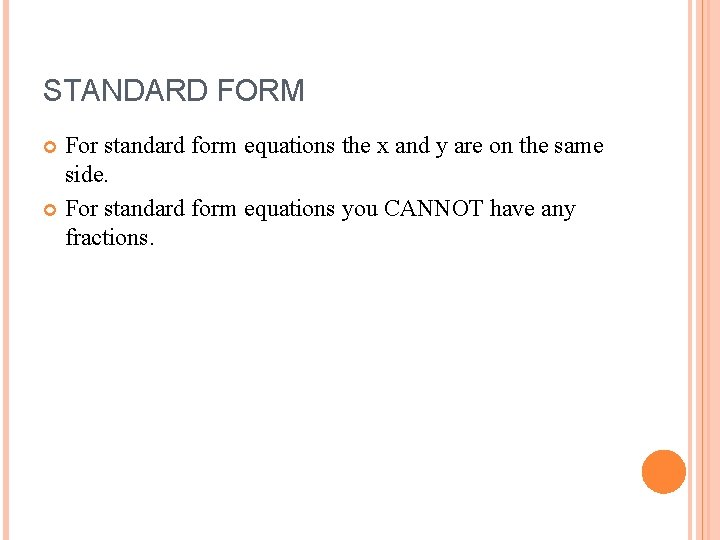STANDARD FORM For standard form equations the x and y are on the same side. For standard form equations you CANNOT have any fractions.STANDARD FORM For standard form equations the x and y are on the same side. For standard form equations you CANNOT have any fractions. For standard form equations your x value HAS to be POSITIVE.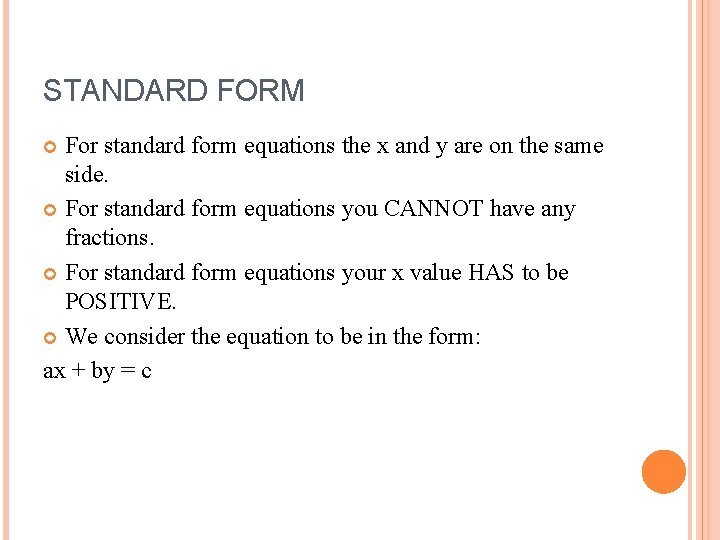STANDARD FORM For standard form equations the x and y are on the same side. For standard form equations you CANNOT have any fractions. For standard form equations your x value HAS to be POSITIVE. We consider the equation to be in the form: ax + by = c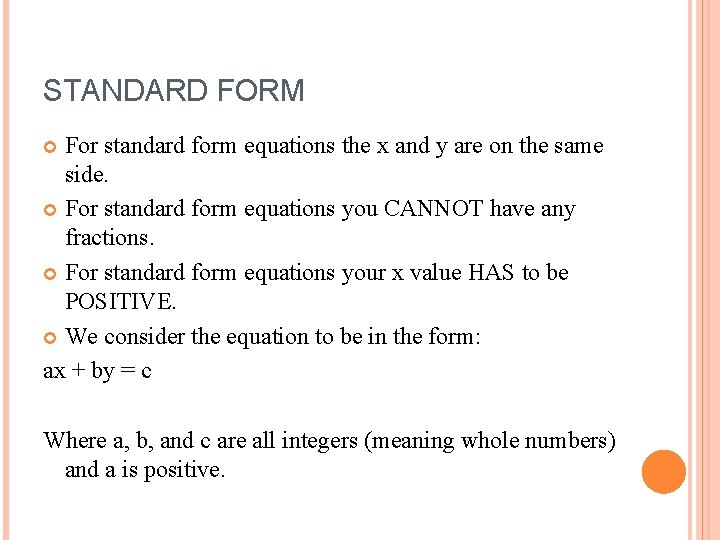STANDARD FORM For standard form equations the x and y are on the same side. For standard form equations you CANNOT have any fractions. For standard form equations your x value HAS to be POSITIVE. We consider the equation to be in the form: ax + by = c Where a, b, and c are all integers (meaning whole numbers) and a is positive.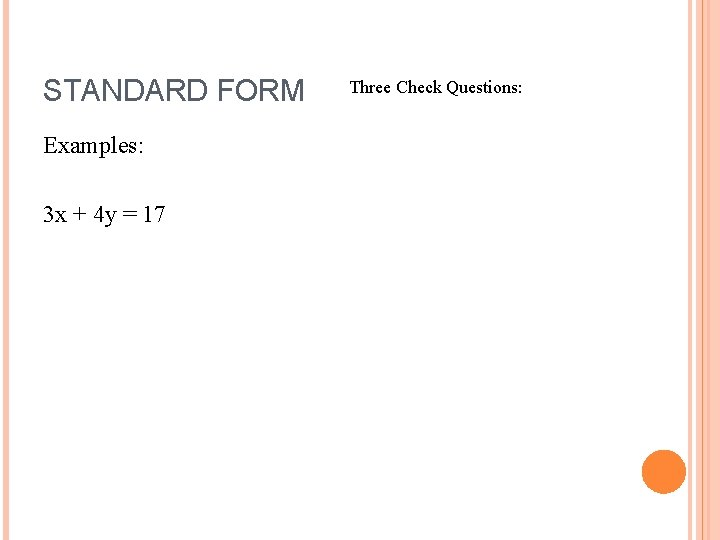STANDARD FORM Examples: 3 x + 4 y = 17 Three Check Questions: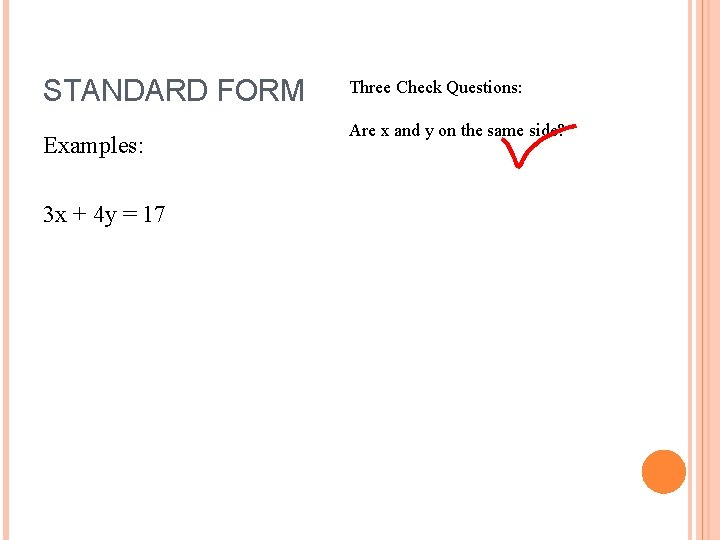STANDARD FORM Examples: 3 x + 4 y = 17 Three Check Questions: Are x and y on the same side?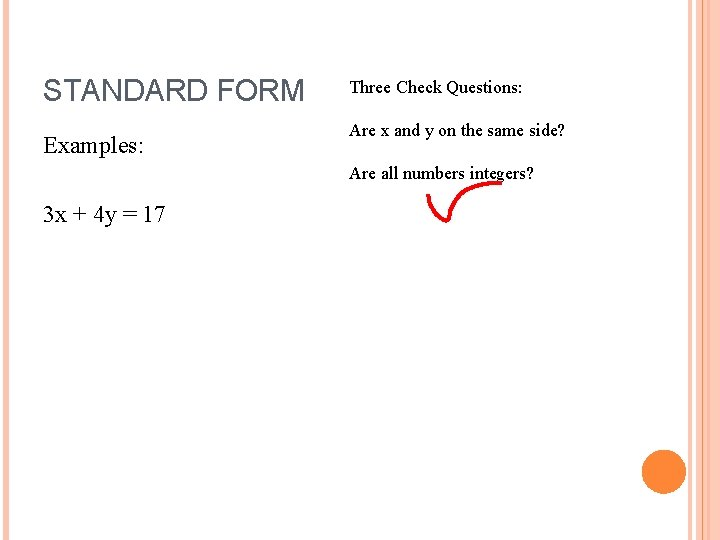STANDARD FORM Examples: Three Check Questions: Are x and y on the same side? Are all numbers integers? 3 x + 4 y = 17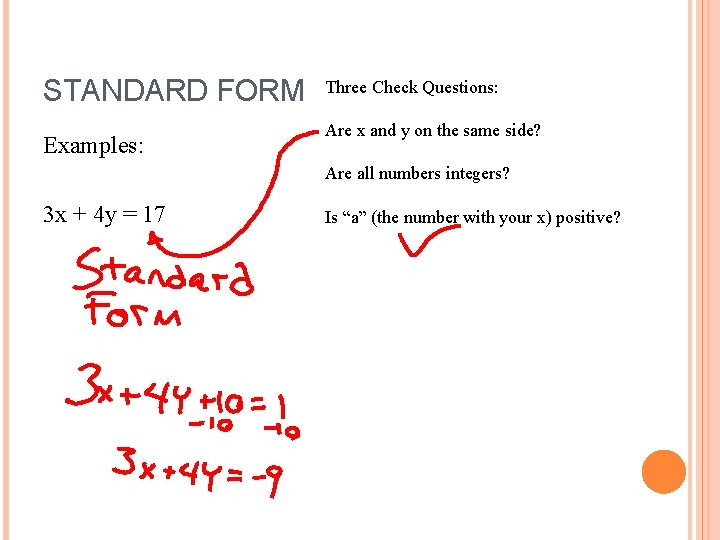STANDARD FORM Examples: Three Check Questions: Are x and y on the same side? Are all numbers integers? 3 x + 4 y = 17 Is “a” (the number with your x) positive?STANDARD FORM Examples: 1/2 x + 3 y = 10 Three Check Questions:STANDARD FORM Examples: 1/2 x + 3 y = 10 Three Check Questions: Are x and y on the same side?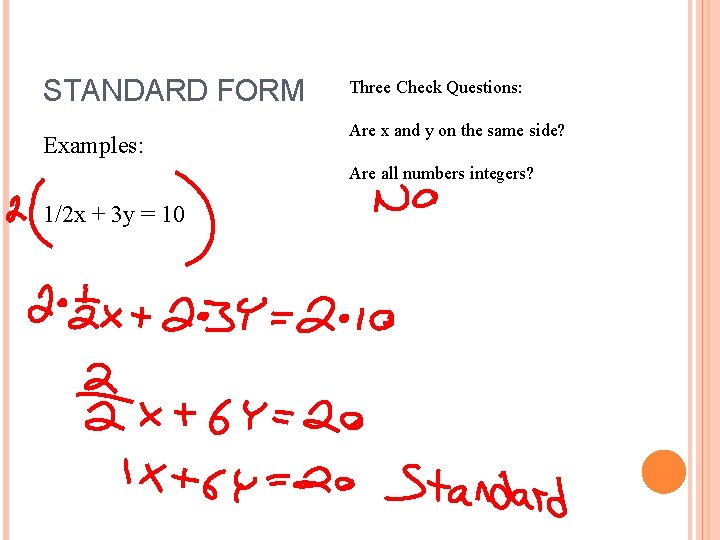STANDARD FORM Examples: Three Check Questions: Are x and y on the same side? Are all numbers integers? 1/2 x + 3 y = 10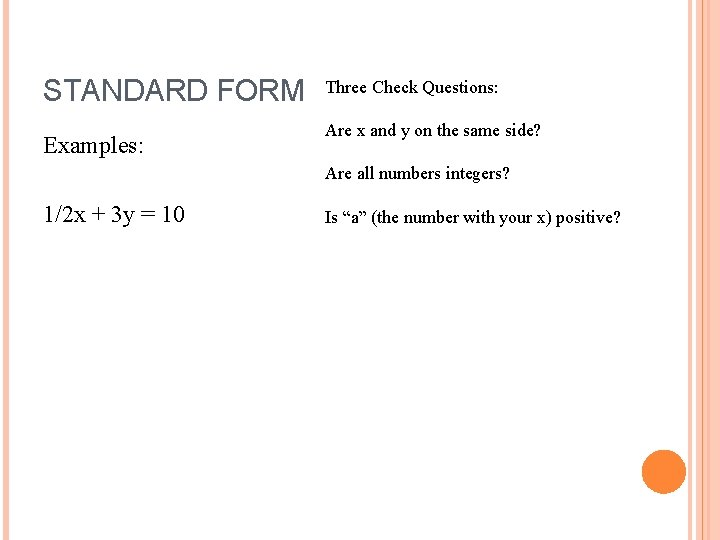STANDARD FORM Examples: Three Check Questions: Are x and y on the same side? Are all numbers integers? 1/2 x + 3 y = 10 Is “a” (the number with your x) positive?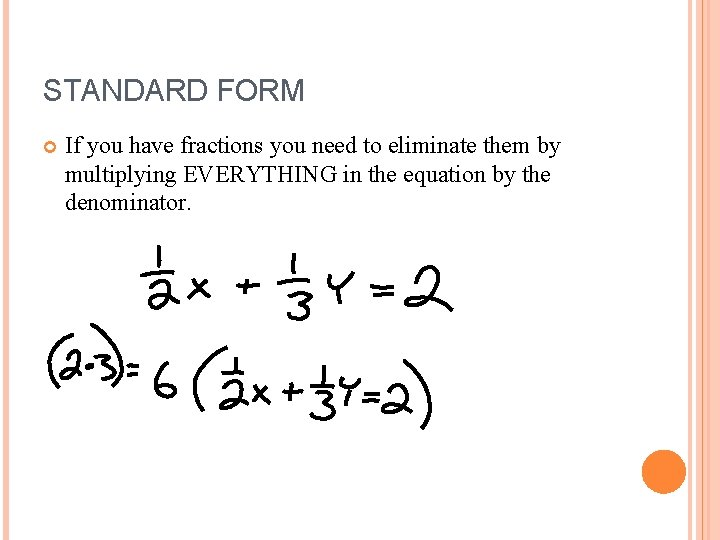STANDARD FORM If you have fractions you need to eliminate them by multiplying EVERYTHING in the equation by the denominator.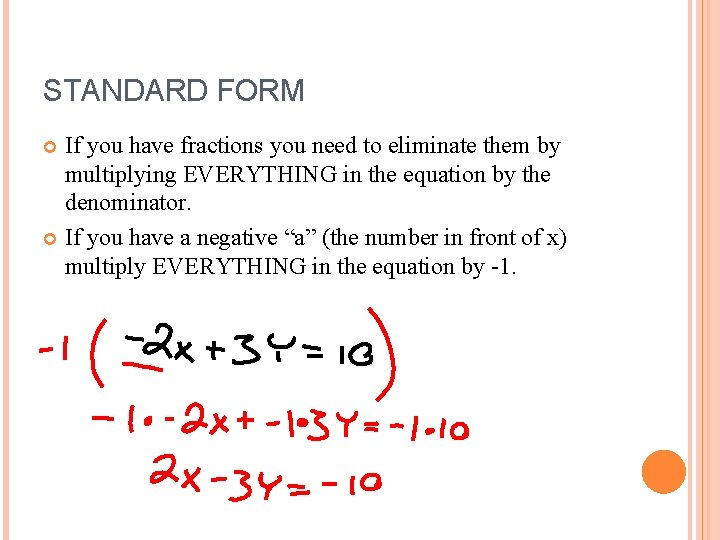STANDARD FORM If you have fractions you need to eliminate them by multiplying EVERYTHING in the equation by the denominator. If you have a negative “a” (the number in front of x) multiply EVERYTHING in the equation by -1.STANDARD FORM If you have fractions you need to eliminate them by multiplying EVERYTHING in the equation by the denominator. If you have a negative “a” (the number in front of x) multiply EVERYTHING in the equation by -1. If x and y are not on the same side, bring them to the same side by eliminating one using addition or subtraction.